### 纳智捷 大7 MPV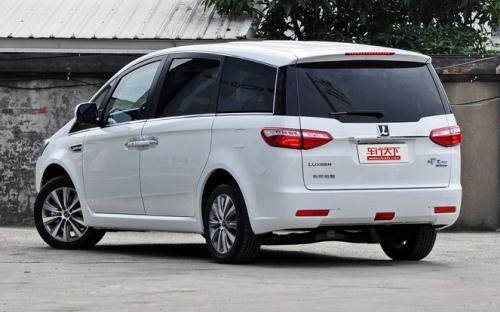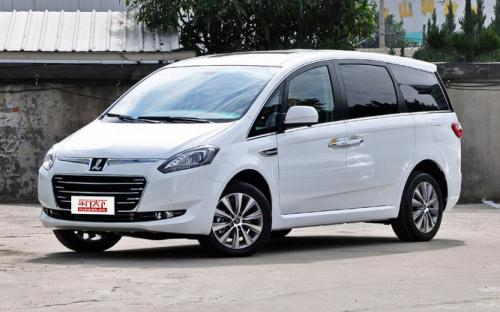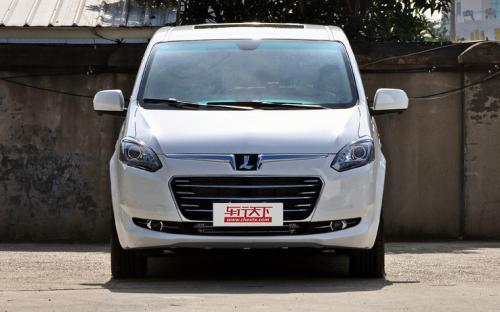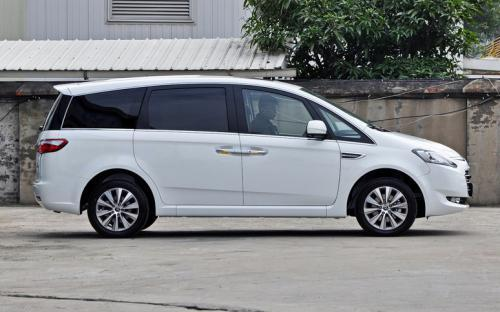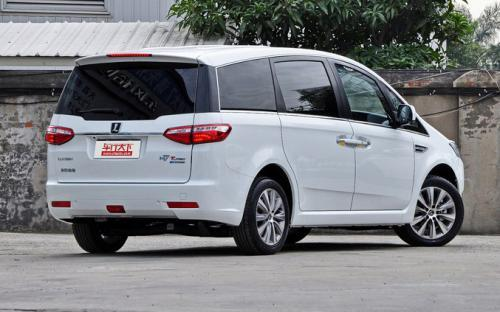0 种颜色可选2016款最低售价：16.98 万元起

4845(mm)1876(mm)1768(mm)##### 配置亮点：
• 胎压监测装置

• ISOFIX儿童座椅接口

• 车身稳定控制(ESC/ESP/DSC等)

• 电动天窗

• 定速巡航

• 后倒车雷达

• 真皮座椅

• GPS导航系统

• 氙气大灯

• 后视镜加热

• 提交
2016款 2.2T 智享型 (119张)
• 2016款 2.2T 智享型 (119张)
• 2016款 2.0T 豪华型 (150张)
• 纳智捷 大7 MPV 绕车实拍• 纳智捷 大7 MPV 在售车型

排量 车型 厂商指导价 本地最低报价 购车工具
2.0T
2.0T 舒享型 6挡手自一体
16.98万
2.0T 豪华型 6挡手自一体
17.98万
2.2T 智享型 6挡手自一体
21.98万
2.2T 旗舰型 左移对比 6挡手自一体
24.98万
2.2T
2.0T 舒享型 6挡手自一体
16.98万
2.0T 豪华型 6挡手自一体
17.98万
2.2T 智享型 6挡手自一体
21.98万
2.2T 旗舰型 左移对比 6挡手自一体
24.98万

纳智捷 大7 MPV 经销商

查看更多 >>

### 纳智捷 大7 MPV 动力加速

大7 MPV 0-100公里加速时间分布在 0.0-秒 属于 超跑级

动力级别 加速时间 车型

纳智捷 大7 MPV 视频

纳智捷 大7 MPV 新闻资讯

# 2016款纳智捷大7 MPV上市 售16.98-24.98万

国产新车 超过9537次关注

3月4日，纳智捷官方宣布，新款大7MPVECOHYPER正式上市，售价为16.98-24.98万元，目前展车已到店。该车采用了与原版相同的外观设计，并且增加了自动启/停等科技配置。

# 纳智捷大7 MPV七座车实用度大调查

车系文章 超过2635次关注

继上回GL8与艾力绅这类较大型的MPV空间深度调查后，今天我们对上市一段时间但消费者不太了解的纳智捷大7MPV做新一期的7座MPV空间深度调查，与之前新佳乐这类紧凑型...

# 激烈竞争中求生 试驾纳智捷 大7 MPV

试驾评测 超过4355次关注

从“不见天日”的首都北京来到清新宜人的厦门，这种畅快的感觉就如同从一辆空间局促的车辆中一头扎进了纳智捷大7MPV的怀抱。不过在这种短暂的幸福感消退之后，我们...

# 纳智捷大7 MPV购车手册 行政版更胜一筹

专业导购 超过3926次关注

继纳智捷推出首款MPV车型MASTERCEO后，在今年的4月份，厂家推出了第二款MPV车型——纳智捷大7MPV。就外观而言，你可以看作是MASTERCEO的七座版本车型，在配置上，纳...

# 东风裕隆大7 MPV配置曝光 即将上市

车系文章 超过2018次关注

日前，我们从东风裕隆官方获得了大7MPV车型的配置信息。这款车的售价已经在2013上海国际车展上正式公布，上市之后共有五款车型，预计上市时间为5月25日。

# 大7 MPV购车送1万礼包 少量现车销售

车系文章 超过1954次关注

近日，从河南东安纳智捷生活馆了解到，大7MPV店内少量现车销售，购车送1万元装饰大礼包（太阳膜、原厂脚垫、VIP贵宾卡、20L油），再送2年4次的常规保养。

# 大7 MPV亮相上海车展 售价19.8万起

车系文章 超过2004次关注

4月20日，上海车展正式拉开了帷幕，东风裕隆在本次车展上公布了大7MPV的售价，这款车即将在今年5月份正式上市。大7MPV共五款车型，售价区间为19.8-27.8万元。

猜你喜欢

﻿
• 快速找车
• 选择品牌
• 选择品牌
• A  奥迪
• A  阿斯顿·马丁
• A  阿尔法·罗密欧
• B  宝沃
• B  布加迪
• B  巴博斯
• B  保时捷
• B  宾利
• B  奔驰
• B  宝马
• B  本田
• B  别克
• B  标致
• B  比亚迪
• B  宝骏
• B  北汽制造
• B  北汽新能源
• B  北汽幻速
• B  北汽威旺
• B  北京汽车
• B  奔腾
• B  北汽绅宝
• C  长安
• C  长安商用
• C  长城
• C  昌河
• D  大众
• D  道奇
• D  DS
• D  东南
• D  东风风神
• D  东风风行
• D  东风小康
• D  东风风度
• D  东风
• F  福特
• F  丰田
• F  菲亚特
• F  法拉利
• F  福田
• F  福迪
• F  福汽启腾
• G  观致
• G  广汽传祺
• G  广汽吉奥
• G  GMC
• H  红旗
• H  汉腾汽车
• H  哈弗
• H  哈飞
• H  海格
• H  海马
• H  华颂
• H  黄海
• H  华泰
• H  恒天
• J  吉利汽车
• J  捷豹
• J  Jeep
• J  江淮
• J  江铃
• J  金杯
• J  九龙
• J  金旅
• K  凯翼
• K  凯迪拉克
• K  克莱斯勒
• K  科尼塞克
• K  卡威
• K  开瑞
• L  路虎
• L  林肯
• L  劳斯莱斯
• L  兰博基尼
• L  雷克萨斯
• L  铃木
• L  雷诺
• L  理念
• L  力帆
• L  莲花汽车
• L  猎豹
• L  路特斯
• L  陆风
• M  马自达
• M  MG
• M  MINI
• M  玛莎拉蒂
• M  摩根
• M  迈凯轮
• N  纳智捷
• O  欧宝
• O  讴歌
• O  欧朗
• Q  奇瑞
• Q  起亚
• Q  启辰
• R  日产
• R  荣威
• R  瑞麒
• S  三菱
• S  斯威汽车
• S  萨博
• S  smart
• S  斯柯达
• S  斯巴鲁
• S  思铭
• S  双龙
• S  上汽大通
• S  双环
• T  特斯拉
• T  腾势
• W  沃尔沃
• W  五菱汽车
• W  五十铃
• W  威兹曼
• W  威麟
• X  现代
• X  雪佛兰
• X  雪铁龙
• X  西雅特
• Y  一汽
• Y  英菲尼迪
• Y  英致
• Y  依维柯
• Y  野马汽车
• Y  永源
• Z  众泰
• Z  中华
• Z  中兴
• Z  知豆
• 选择车系
• 选择车系
• 车型对比
• 选择品牌
• 选择品牌
• A  奥迪
• A  阿斯顿·马丁
• A  阿尔法·罗密欧
• B  宝沃
• B  布加迪
• B  巴博斯
• B  保时捷
• B  宾利
• B  奔驰
• B  宝马
• B  本田
• B  别克
• B  标致
• B  比亚迪
• B  宝骏
• B  北汽制造
• B  北汽新能源
• B  北汽幻速
• B  北汽威旺
• B  北京汽车
• B  奔腾
• B  北汽绅宝
• C  长安
• C  长安商用
• C  长城
• C  昌河
• D  大众
• D  道奇
• D  DS
• D  东南
• D  东风风神
• D  东风风行
• D  东风小康
• D  东风风度
• D  东风
• F  福特
• F  丰田
• F  菲亚特
• F  法拉利
• F  福田
• F  福迪
• F  福汽启腾
• G  观致
• G  广汽传祺
• G  广汽吉奥
• G  GMC
• H  红旗
• H  汉腾汽车
• H  哈弗
• H  哈飞
• H  海格
• H  海马
• H  华颂
• H  黄海
• H  华泰
• H  恒天
• J  吉利汽车
• J  捷豹
• J  Jeep
• J  江淮
• J  江铃
• J  金杯
• J  九龙
• J  金旅
• K  凯翼
• K  凯迪拉克
• K  克莱斯勒
• K  科尼塞克
• K  卡威
• K  开瑞
• L  路虎
• L  林肯
• L  劳斯莱斯
• L  兰博基尼
• L  雷克萨斯
• L  铃木
• L  雷诺
• L  理念
• L  力帆
• L  莲花汽车
• L  猎豹
• L  路特斯
• L  陆风
• M  马自达
• M  MG
• M  MINI
• M  玛莎拉蒂
• M  摩根
• M  迈凯轮
• N  纳智捷
• O  欧宝
• O  讴歌
• O  欧朗
• Q  奇瑞
• Q  起亚
• Q  启辰
• R  日产
• R  荣威
• R  瑞麒
• S  三菱
• S  斯威汽车
• S  萨博
• S  smart
• S  斯柯达
• S  斯巴鲁
• S  思铭
• S  双龙
• S  上汽大通
• S  双环
• T  特斯拉
• T  腾势
• W  沃尔沃
• W  五菱汽车
• W  五十铃
• W  威兹曼
• W  威麟
• X  现代
• X  雪佛兰
• X  雪铁龙
• X  西雅特
• Y  一汽
• Y  英菲尼迪
• Y  英致
• Y  依维柯
• Y  野马汽车
• Y  永源
• Z  众泰
• Z  中华
• Z  中兴
• Z  知豆
• 选择车系
• 选择车系
• 选择车型
• 选择车型
• 意见反馈